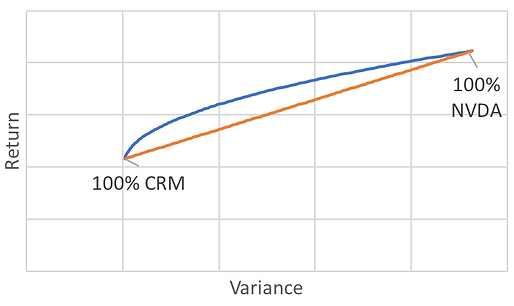#### Modern Portfolio Theory

The Modern Portfolio Theory was invented by Harry Markowitz and explains how a portfolio of stocks can be optimised to achieve a maximum return, at a given risk level; or vice versa, how to minimise risk at a given level of return. The theory is based on historical returns and uses the covariance to achieve its goal.

A stock portfolio's return is calculated by the weighted average of the stocks it contains. If portfolio ABCD consists of stock A (10%), B (10%), C (40%) and D (40%), and the returns of the stocks are 2%, 4%, 6% and 8% respectively, the portfolio's overall return will be:

(10% x 2%) + (10% x 4%) + (40% x 6%) + (40% x 8%) = 6,2%

STEP 1 - returns

Step 2 -
Variances and covariances

A stock's risk is often referred to as its volatility, and thus refers to the amount by which the stock has swung up and down in its past. The higher the swings (in past returns), the higher the volatility, the higher the variance, the higher the risk. The portfolio variance, however, is not calculated like the portfolio returns. It is not the weighted average.

That is because stocks A, B, C and D are correlated. That means that if stock A goes down drastically, statistically spoken there is quite a chance that stocks B, C, D will not go down as much as A, and thus decreasing the overall risk of the portfolio. This is as true for downward movements, as it is for upward movements.

Let's have a look at how this is visually represented with two stocks: Nvidia (NVDA) and Salesforce (CRM):The orange line represents the portfolio risk/return as if there were no correlation to be found between both stocks. On the left a portfolio consisting of 100% Salesforce, on the right a portfolio consisting of 100% Nvidia. The blue line represents the actual portfolio risk/return by using the covariance, based on the last 6 months of 2017 of historical return of both stocks.

Now if we upscale the number of stocks, like in the image below, we get a whole different picture. The blue dots represent the individual stocks of a chosen investment universe with their respective risk (average daily volatility) versus return (average daily returns), in 2017.

The red dots represent optimal portfolios according to the Modern Portfolio Theory, and as such the optimal combination of the underlying stocks (blue dots), with respectively target annual returns of 20%, 30%, 40%, ... Thanks to the covariance between the different stocks, significant risk optimisations are achieved at fixed return levels.

I applied a maximal allocation in each of the calculated portfolios in this example at 10%. The red line represents the efficient frontier, and is a combination of all portfolios for which risk/return is optimised. This frontier has been calculated by using the Excel non-linear Solver.

STEP 3 -
Full scale portfoliosConclusions

An optimal portfolio is what you want to invest in as a diversified investor, because at that point, you get either the minimal volatility (or risk) for a target return, either a maximum return for a given volatility (or risk level). The volatility of individual assets offset on another (This effect can be calculated through covariance) and as a result, risk is minimised, while equal returns can be achieved.